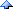# 77家的会客厅2010

PowerShell将配置文件转换为字典(hash table)

```#this is a test conf file
[SectionName1]
name=abc
id=123
[dbinfo]
db=localhost
name=testdb
user=root
```

```function global:Conf2Dict{
param([string]\$confFile)
if(test-path \$confFile -pathType Leaf)
{
\$dict= @{}
foreach (\$line in (Get-Content \$confFile)) {
\$lin = \$line.Trim()
if(!\$lin.startsWith("#") -and \$lin -ne (\$null -or "")) {
if(\$lin -Match "^\[\w+\]\$" -and \$lin.Length -gt 2){
\$k = \$lin.Substring(1,\$lin.Length-2)
\$dict[\$k] = @{}
}else{
\$arrPath = \$lin.Split("=")
\$nam = \$arrPath.Trim()
\$var = \$arrPath.Trim()
\$dict[\$k][\$nam] = \$var
}
}
}
if(\$dict.Count -ge 1){
return \$dict
}else{
return \$null
}
}else{
return \$null
}
}
```(10/24) 我已经没办法专注于一件事了！

[2006/11/10]也发wallop和gmail邀请
[2005/11/10]浦发银行太不与时俱进了